# Mean State

Period Mean (original grids) [W/m2]
Model Period Mean (intersection) [W/m2]
Model Period Mean (complement) [W/m2]
Benchmark Period Mean (intersection) [W/m2]
Benchmark Period Mean (complement) [W/m2]
Bias [W/m2]
RMSE [W/m2]
Phase Shift [months]
Bias Score 
RMSE Score 
Seasonal Cycle Score 
Spatial Distribution Score 
Interannual Variability Score 
Overall Score 
Benchmark [-] 31.3
CLM5PHSOFF [-] 34.7 34.4 0.00 32.3 12.4 2.23 8.02 1.94 0.37 0.32 0.72 0.93 0.72 0.56
CLM5PHSON [-] 33.7 33.4 0.00 32.3 12.4 1.78 7.73 1.92 0.39 0.33 0.72 0.92 0.70 0.57
Period Mean (original grids) [W/m2]
Model Period Mean (intersection) [W/m2]
Model Period Mean (complement) [W/m2]
Benchmark Period Mean (intersection) [W/m2]
Benchmark Period Mean (complement) [W/m2]
Bias [W/m2]
RMSE [W/m2]
Phase Shift [months]
Bias Score 
RMSE Score 
Seasonal Cycle Score 
Spatial Distribution Score 
Interannual Variability Score 
Overall Score 
Benchmark [-] 44.7
CLM5PHSOFF [-] 46.3 46.8 0.00 45.1 13.9 1.52 9.10 0.947 0.53 0.32 0.88 0.98 0.77 0.63
CLM5PHSON [-] 45.2 45.7 0.00 45.1 13.9 0.386 8.71 0.894 0.53 0.34 0.89 0.97 0.72 0.63
Period Mean (original grids) [W/m2]
Model Period Mean (intersection) [W/m2]
Model Period Mean (complement) [W/m2]
Benchmark Period Mean (intersection) [W/m2]
Benchmark Period Mean (complement) [W/m2]
Bias [W/m2]
RMSE [W/m2]
Phase Shift [months]
Bias Score 
RMSE Score 
Seasonal Cycle Score 
Spatial Distribution Score 
Interannual Variability Score 
Overall Score 
Benchmark [-] 33.4
CLM5PHSOFF [-] 36.8 36.8 0.00 34.3 15.0 2.44 9.00 1.51 0.56 0.38 0.79 0.93 0.75 0.63
CLM5PHSON [-] 36.3 36.3 0.00 34.3 15.0 2.16 8.43 1.35 0.55 0.41 0.82 0.90 0.71 0.63
Period Mean (original grids) [W/m2]
Model Period Mean (intersection) [W/m2]
Model Period Mean (complement) [W/m2]
Benchmark Period Mean (intersection) [W/m2]
Benchmark Period Mean (complement) [W/m2]
Bias [W/m2]
RMSE [W/m2]
Phase Shift [months]
Bias Score 
RMSE Score 
Seasonal Cycle Score 
Spatial Distribution Score 
Interannual Variability Score 
Overall Score 
Benchmark [-] 40.8
CLM5PHSOFF [-] 56.0 36.7 0.00 41.2 35.6 -4.39 21.6 0.260 0.83 0.66 0.98 0.96 0.67 0.79
CLM5PHSON [-] 33.3 33.3 0.00 41.2 35.6 -7.13 23.8 0.214 0.81 0.64 0.99 0.92 0.66 0.78
Period Mean (original grids) [W/m2]
Model Period Mean (intersection) [W/m2]
Model Period Mean (complement) [W/m2]
Benchmark Period Mean (intersection) [W/m2]
Benchmark Period Mean (complement) [W/m2]
Bias [W/m2]
RMSE [W/m2]
Phase Shift [months]
Bias Score 
RMSE Score 
Seasonal Cycle Score 
Spatial Distribution Score 
Interannual Variability Score 
Overall Score 
Benchmark [-] 17.7
CLM5PHSOFF [-] 28.7 28.6 0.00 19.3 12.3 9.27 10.8 1.52 0.27 0.36 0.77 0.43 0.69 0.48
CLM5PHSON [-] 27.5 27.5 0.00 19.3 12.3 8.88 10.5 1.50 0.28 0.37 0.77 0.36 0.69 0.47
Period Mean (original grids) [W/m2]
Model Period Mean (intersection) [W/m2]
Model Period Mean (complement) [W/m2]
Benchmark Period Mean (intersection) [W/m2]
Benchmark Period Mean (complement) [W/m2]
Bias [W/m2]
RMSE [W/m2]
Phase Shift [months]
Bias Score 
RMSE Score 
Seasonal Cycle Score 
Spatial Distribution Score 
Interannual Variability Score 
Overall Score 
Benchmark [-] 28.1
CLM5PHSOFF [-] 29.5 29.5 0.00 28.2 21.3 1.27 12.9 0.212 0.77 0.59 0.99 0.99 0.74 0.78
CLM5PHSON [-] 25.2 25.1 0.00 28.2 21.3 -3.04 11.8 0.270 0.79 0.62 0.98 0.98 0.70 0.78
Period Mean (original grids) [W/m2]
Model Period Mean (intersection) [W/m2]
Model Period Mean (complement) [W/m2]
Benchmark Period Mean (intersection) [W/m2]
Benchmark Period Mean (complement) [W/m2]
Bias [W/m2]
RMSE [W/m2]
Phase Shift [months]
Bias Score 
RMSE Score 
Seasonal Cycle Score 
Spatial Distribution Score 
Interannual Variability Score 
Overall Score 
Benchmark [-] 35.8
CLM5PHSOFF [-] 35.0 35.0 0.00 36.5 15.2 -1.53 11.1 0.929 0.69 0.54 0.88 0.88 0.69 0.70
CLM5PHSON [-] 35.1 35.1 0.00 36.5 15.2 -2.09 11.0 0.920 0.69 0.54 0.88 0.89 0.68 0.70
Period Mean (original grids) [W/m2]
Model Period Mean (intersection) [W/m2]
Model Period Mean (complement) [W/m2]
Benchmark Period Mean (intersection) [W/m2]
Benchmark Period Mean (complement) [W/m2]
Bias [W/m2]
RMSE [W/m2]
Phase Shift [months]
Bias Score 
RMSE Score 
Seasonal Cycle Score 
Spatial Distribution Score 
Interannual Variability Score 
Overall Score 
Benchmark [-] 29.2
CLM5PHSOFF [-] 33.3 33.2 0.00 30.4 12.7 2.70 9.72 1.13 0.54 0.41 0.87 0.82 0.70 0.63
CLM5PHSON [-] 33.2 33.2 0.00 30.4 12.7 2.27 9.43 1.13 0.55 0.43 0.87 0.80 0.67 0.63
Period Mean (original grids) [W/m2]
Model Period Mean (intersection) [W/m2]
Model Period Mean (complement) [W/m2]
Benchmark Period Mean (intersection) [W/m2]
Benchmark Period Mean (complement) [W/m2]
Bias [W/m2]
RMSE [W/m2]
Phase Shift [months]
Bias Score 
RMSE Score 
Seasonal Cycle Score 
Spatial Distribution Score 
Interannual Variability Score 
Overall Score 
Benchmark [-] 36.1
CLM5PHSOFF [-] 32.7 32.5 0.00 36.1 36.1 -3.50 19.6 0.174 0.87 0.68 0.99 0.86 0.70 0.80
CLM5PHSON [-] 31.4 31.3 0.00 36.1 36.1 -4.28 20.1 0.202 0.87 0.68 0.99 0.86 0.70 0.80
Period Mean (original grids) [W/m2]
Model Period Mean (intersection) [W/m2]
Model Period Mean (complement) [W/m2]
Benchmark Period Mean (intersection) [W/m2]
Benchmark Period Mean (complement) [W/m2]
Bias [W/m2]
RMSE [W/m2]
Phase Shift [months]
Bias Score 
RMSE Score 
Seasonal Cycle Score 
Spatial Distribution Score 
Interannual Variability Score 
Overall Score 
Benchmark [-] 36.6
CLM5PHSOFF [-] 39.1 39.2 0.00 38.5 12.8 0.755 9.14 0.682 0.62 0.56 0.93 0.82 0.71 0.70
CLM5PHSON [-] 39.4 39.4 0.00 38.5 12.8 0.0558 9.10 0.622 0.62 0.56 0.94 0.80 0.69 0.70
Period Mean (original grids) [W/m2]
Model Period Mean (intersection) [W/m2]
Model Period Mean (complement) [W/m2]
Benchmark Period Mean (intersection) [W/m2]
Benchmark Period Mean (complement) [W/m2]
Bias [W/m2]
RMSE [W/m2]
Phase Shift [months]
Bias Score 
RMSE Score 
Seasonal Cycle Score 
Spatial Distribution Score 
Interannual Variability Score 
Overall Score 
Benchmark [-] 19.6
CLM5PHSOFF [-] 22.7 22.7 0.00 20.5 10.1 2.16 9.06 0.916 0.70 0.56 0.88 0.87 0.68 0.71
CLM5PHSON [-] 22.3 22.3 0.00 20.5 10.1 1.68 8.97 0.854 0.69 0.56 0.90 0.84 0.67 0.70
Period Mean (original grids) [W/m2]
Model Period Mean (intersection) [W/m2]
Model Period Mean (complement) [W/m2]
Benchmark Period Mean (intersection) [W/m2]
Benchmark Period Mean (complement) [W/m2]
Bias [W/m2]
RMSE [W/m2]
Phase Shift [months]
Bias Score 
RMSE Score 
Seasonal Cycle Score 
Spatial Distribution Score 
Interannual Variability Score 
Overall Score 
Benchmark [-] 24.0
CLM5PHSOFF [-] 42.9 44.9 0.00 44.8 14.0 0.0948 12.9 0.792 0.65 0.54 0.90 0.99 0.70 0.72
CLM5PHSON [-] 44.9 44.9 0.00 44.8 14.0 -0.748 12.7 0.768 0.65 0.55 0.91 0.99 0.69 0.72
Period Mean (original grids) [W/m2]
Model Period Mean (intersection) [W/m2]
Model Period Mean (complement) [W/m2]
Benchmark Period Mean (intersection) [W/m2]
Benchmark Period Mean (complement) [W/m2]
Bias [W/m2]
RMSE [W/m2]
Phase Shift [months]
Bias Score 
RMSE Score 
Seasonal Cycle Score 
Spatial Distribution Score 
Interannual Variability Score 
Overall Score 
Benchmark [-] 72.5
CLM5PHSOFF [-] 76.8 76.7 0.00 74.7 16.4 1.93 13.9 0.733 0.59 0.56 0.93 0.98 0.71 0.72
CLM5PHSON [-] 78.5 78.3 0.00 74.7 16.4 1.75 14.0 0.756 0.59 0.56 0.92 0.98 0.70 0.72
Period Mean (original grids) [W/m2]
Model Period Mean (intersection) [W/m2]
Model Period Mean (complement) [W/m2]
Benchmark Period Mean (intersection) [W/m2]
Benchmark Period Mean (complement) [W/m2]
Bias [W/m2]
RMSE [W/m2]
Phase Shift [months]
Bias Score 
RMSE Score 
Seasonal Cycle Score 
Spatial Distribution Score 
Interannual Variability Score 
Overall Score 
Benchmark [-] 46.1
CLM5PHSOFF [-] 39.5 39.5 0.00 46.3 43.2 -6.74 22.9 0.180 0.84 0.67 0.99 0.92 0.68 0.79
CLM5PHSON [-] 37.3 37.3 0.00 46.3 43.2 -7.82 24.1 0.184 0.83 0.66 0.99 0.96 0.67 0.79
Period Mean (original grids) [W/m2]
Model Period Mean (intersection) [W/m2]
Model Period Mean (complement) [W/m2]
Benchmark Period Mean (intersection) [W/m2]
Benchmark Period Mean (complement) [W/m2]
Bias [W/m2]
RMSE [W/m2]
Phase Shift [months]
Bias Score 
RMSE Score 
Seasonal Cycle Score 
Spatial Distribution Score 
Interannual Variability Score 
Overall Score 
Benchmark [-] 26.3
CLM5PHSOFF [-] 34.1 34.1 0.00 28.9 13.2 5.01 11.1 0.814 0.43 0.47 0.90 0.64 0.71 0.60
CLM5PHSON [-] 33.9 33.9 0.00 28.9 13.2 4.55 10.7 0.760 0.44 0.49 0.91 0.62 0.69 0.61
Period Mean (original grids) [W/m2]
Model Period Mean (intersection) [W/m2]
Model Period Mean (complement) [W/m2]
Benchmark Period Mean (intersection) [W/m2]
Benchmark Period Mean (complement) [W/m2]
Bias [W/m2]
RMSE [W/m2]
Phase Shift [months]
Bias Score 
RMSE Score 
Seasonal Cycle Score 
Spatial Distribution Score 
Interannual Variability Score 
Overall Score 
Benchmark [-] 26.9
CLM5PHSOFF [-] 28.4 28.4 0.00 27.6 12.3 0.754 8.91 1.21 0.71 0.52 0.82 0.88 0.68 0.69
CLM5PHSON [-] 27.6 27.5 0.00 27.6 12.3 -0.305 8.02 1.11 0.72 0.55 0.84 0.83 0.67 0.69
Period Mean (original grids) [W/m2]
Model Period Mean (intersection) [W/m2]
Model Period Mean (complement) [W/m2]
Benchmark Period Mean (intersection) [W/m2]
Benchmark Period Mean (complement) [W/m2]
Bias [W/m2]
RMSE [W/m2]
Phase Shift [months]
Bias Score 
RMSE Score 
Seasonal Cycle Score 
Spatial Distribution Score 
Interannual Variability Score 
Overall Score 
Benchmark [-] 26.3
CLM5PHSOFF [-] 27.0 27.0 0.00 26.9 10.9 0.228 13.8 0.325 0.69 0.54 0.96 0.98 0.66 0.73
CLM5PHSON [-] 23.6 23.7 0.00 26.9 10.9 -3.27 12.6 0.410 0.72 0.56 0.96 0.97 0.61 0.73
Period Mean (original grids) [W/m2]
Model Period Mean (intersection) [W/m2]
Model Period Mean (complement) [W/m2]
Benchmark Period Mean (intersection) [W/m2]
Benchmark Period Mean (complement) [W/m2]
Bias [W/m2]
RMSE [W/m2]
Phase Shift [months]
Bias Score 
RMSE Score 
Seasonal Cycle Score 
Spatial Distribution Score 
Interannual Variability Score 
Overall Score 
Benchmark [-] 38.3
CLM5PHSOFF [-] 36.1 36.0 0.00 38.7 12.8 -2.80 10.1 0.977 0.73 0.57 0.86 0.96 0.75 0.74
CLM5PHSON [-] 34.6 34.6 0.00 38.7 12.8 -4.44 10.2 1.05 0.68 0.58 0.85 0.97 0.72 0.73
Period Mean (original grids) [W/m2]
Model Period Mean (intersection) [W/m2]
Model Period Mean (complement) [W/m2]
Benchmark Period Mean (intersection) [W/m2]
Benchmark Period Mean (complement) [W/m2]
Bias [W/m2]
RMSE [W/m2]
Phase Shift [months]
Bias Score 
RMSE Score 
Seasonal Cycle Score 
Spatial Distribution Score 
Interannual Variability Score 
Overall Score 
Benchmark [-] 27.6
CLM5PHSOFF [-] 27.6 27.6 0.00 28.0 11.7 -0.361 5.13 1.19 0.57 0.44 0.85 0.81 0.65 0.63
CLM5PHSON [-] 27.1 27.1 0.00 28.0 11.7 -0.726 5.09 1.15 0.55 0.45 0.86 0.75 0.63 0.61
Period Mean (original grids) [W/m2]
Model Period Mean (intersection) [W/m2]
Model Period Mean (complement) [W/m2]
Benchmark Period Mean (intersection) [W/m2]
Benchmark Period Mean (complement) [W/m2]
Bias [W/m2]
RMSE [W/m2]
Phase Shift [months]
Bias Score 
RMSE Score 
Seasonal Cycle Score 
Spatial Distribution Score 
Interannual Variability Score 
Overall Score 
Benchmark [-] 31.2
CLM5PHSOFF [-] 32.2 32.2 0.00 31.8 11.7 0.390 6.69 1.27 0.69 0.47 0.83 0.88 0.77 0.69
CLM5PHSON [-] 31.6 31.6 0.00 31.8 11.7 -0.285 6.44 1.08 0.67 0.49 0.86 0.80 0.73 0.67
Period Mean (original grids) [W/m2]
Model Period Mean (intersection) [W/m2]
Model Period Mean (complement) [W/m2]
Benchmark Period Mean (intersection) [W/m2]
Benchmark Period Mean (complement) [W/m2]
Bias [W/m2]
RMSE [W/m2]
Phase Shift [months]
Bias Score 
RMSE Score 
Seasonal Cycle Score 
Spatial Distribution Score 
Interannual Variability Score 
Overall Score 
Benchmark [-] 30.1
CLM5PHSOFF [-] 32.4 32.3 0.00 32.0 13.9 0.318 9.95 0.845 0.56 0.52 0.91 0.96 0.72 0.70
CLM5PHSON [-] 33.2 33.2 0.00 32.0 13.9 0.808 9.75 0.826 0.57 0.53 0.91 0.96 0.70 0.70

# Temporally integrated period mean

BENCHMARK MEAN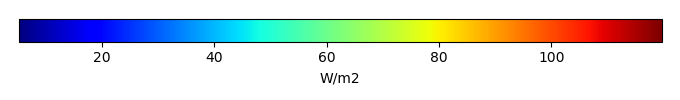MODEL MEANBIAS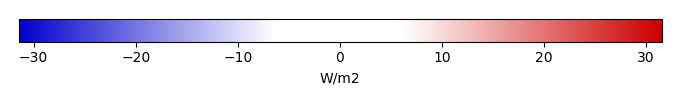BIAS SCORERMSE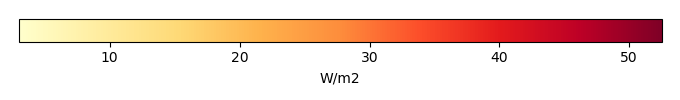RMSE SCOREBENCHMARK INTERANNUAL VARIABILITY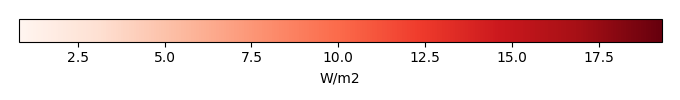MODEL INTERANNUAL VARIABILITYINTERANNUAL VARIABILITY SCOREBENCHMARK MAX MONTHMODEL MAX MONTHDIFFERENCE IN MAX MONTH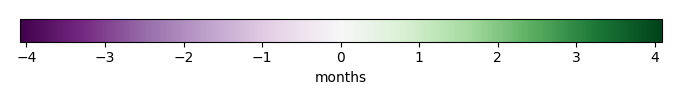SEASONAL CYCLE SCORESPATIAL TAYLOR DIAGRAMMODEL COLORS# Spatially integrated regional mean

MODEL COLORSREGIONAL MEANANNUAL CYCLEMONTHLY ANOMALYANNUAL CYCLE# All Models

BenchmarkCLM5PHSOFFCLM5PHSON# Data Information

creation_date: Thu May 8 23:27:16 PDT 2014

source_file: This product is generated from monthly 1 degree CERES EBAF Radiation observations